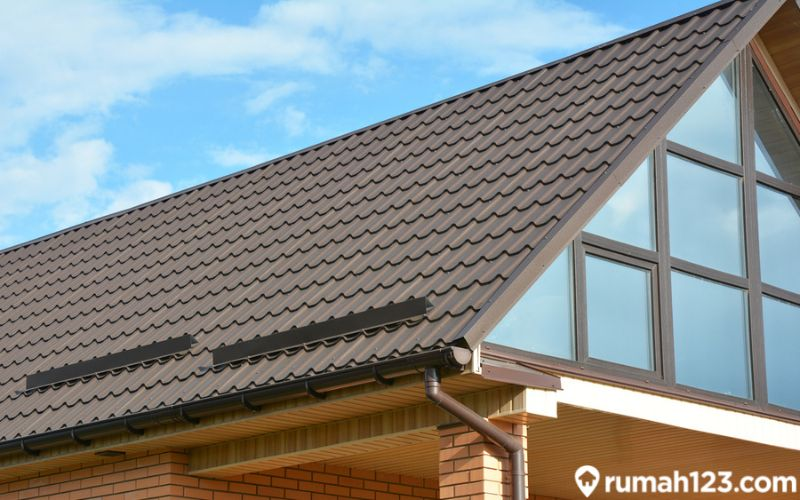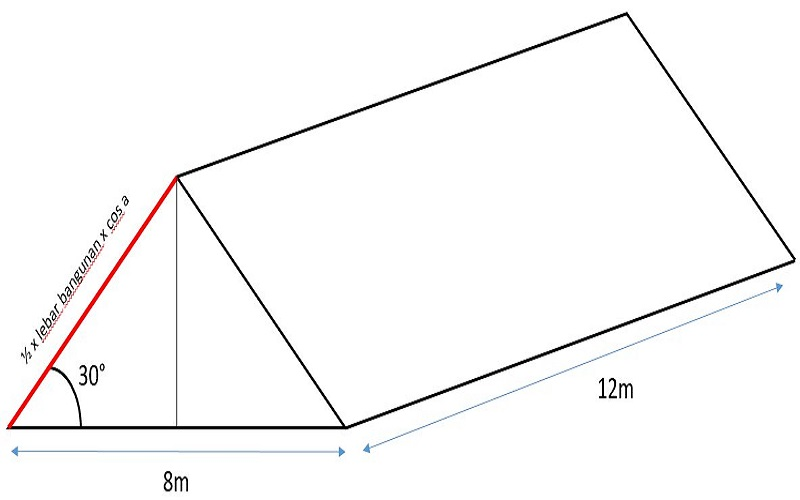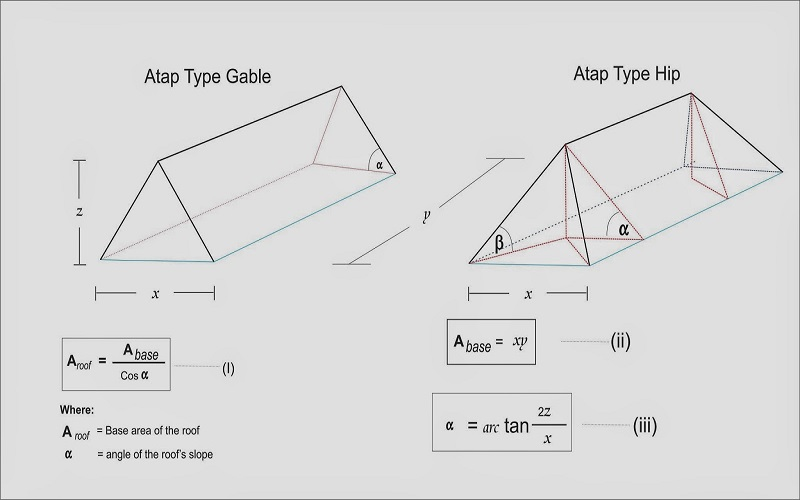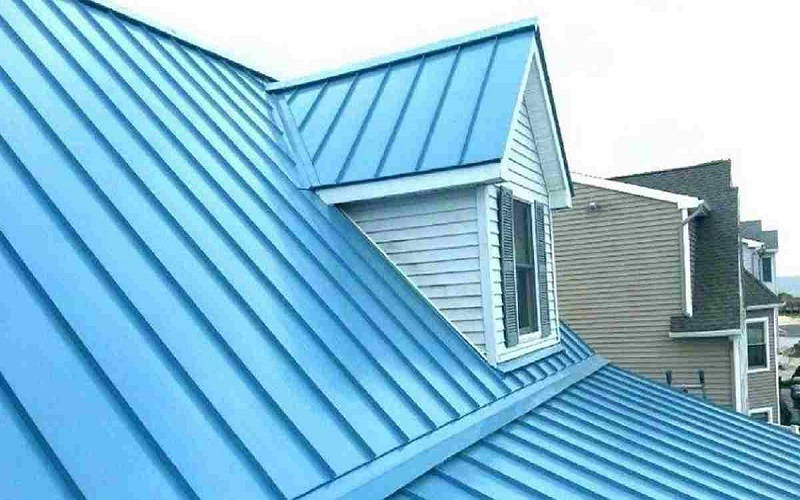Breaking News# How to calculate the correct slope of the roof of a house, it’s easy!

How to calculate the correct slope of the roof of a house, it’s easy!See how to calculate the correct slope of the roof for the complete needs of the building here.

In the determination of a house, all things related to the building must be carefully considered.

Not only the size of the land area and the buildings, the slope of the roof of the house should also be an important concern for renovation needs and others.

The reason is, the roof of the house certainly has a different slope depending on the structure of the house and the construction of the building.

The construction of the roof is a vital part of a building that functions as a protector of the weather, be it the sun, wind or rain.

In addition to protection against the weather, the slope of the roof can also drain rainwater well into the ground so that it enters the ground.

For water drainage and weather resistance to function properly, it is a good idea to calculate the slope of the roof of the house first so that it is more comfortable.

See also  Understand the exhaust fan and how to choose

What are the things you need to calculate so that the roof has a good functional value? Check out the discussion together!

## Knowing the angle of the roof of the houseSource: Bluescopezacs.co.id

The size of the corner of the roof of the house is the ideal limit for the roof truss to cover, so that the water can be well distributed to the ground.

In areas with a tropical climate, the ideal angle of the roof of the house is to have a strong part of about 30 degrees, with details between 22.5 – 30 degrees.

Meanwhile, if the roof of the house you use is clay tile, the angle is between 27.5 – 40 degrees.

You can adjust the angle of the roof of the house according to your needs, so you don’t have leaks or infiltrations.

## How to calculate the slope of the roof of the house well and correctlySource: Civil.uma.ac.id

### 1. Check the half length of the roof of the house

How to calculate the slope of the roof of the first house that you can do is to check the length of the middle of the base of the roof of the house.

That is, you have to measure the length of the side of the roof of the house and then divide by two as necessary.

If the total length of the side of the roof is 10 meters, it means that the number you use is 5 meters.

### 2. Know the height of the roof of the house

Then, you can measure the height of the roof of the house specifically.

See also  7 easy ways to win a warm room

You can measure the limit of the roof of the house at the highest side of the roof seen on the side of the split or dividing the roof of the house.

For example, you can get a ceiling height of 3 meters.

### 3. How to Calculate the Slope of the Roof of a House with Pythagoras

After knowing the control for half the length and height of the roof of the house, how to calculate the slope of the roof of the nearby house is to use the formula pythagoras

The formula is c² = b² + a²,

1. a: is the height of the roof
2. b: is the base of the roof
3. c: is the slope of the roof.

1. Roof slope = Base² + Height²

2. Roof slope = 5² + 32

3. Roof slope = 25 + 9

4. Roof slope = 34

5. Slope of the roof = 5.83 meters

So the slope of the roof of the house from the analysis is 5.83 meters.

This calculation is to find the slope of the roof in meters, not in standard degrees.

## How to Calculate the Slope of the Roof of a House Based on the Standard TypeSource: Harapanrakyat.com

In addition to calculating in meters, you can find the level of slope based on the type with units of degrees.

1. Zinc roof: 15 – 25 degrees.
2. Asphalt roof: 30 – 90 degrees.
3. Concrete roof: 30 – 35 degrees.
4. Metal roof: 25 – 35 degrees.
5. Ceramic roof: 30 degree range.
6. Roof deck: 0 degrees.
7. Glass roof: 2 – 90 degrees.
8. Spandek roof: 5 – 60 degrees.
See also  Again, it offers ROI for villa purchases in Bali

The reference measurement standard must also be readjusted to the structure of the building or the support of the roof, to give a sense of security to the dwelling.

I hope that this information on how to calculate the correct roof of a house is useful for the needs of building a house.

Find interesting information about the property, more about www.lacrymosemedia.com.

Create a dream home with an environmental vision together Mississippi Cluster Tourist City more to www.lacrymosemedia.com and www.lacrymosemedia.comthat certainly #AdaBuatKamu!